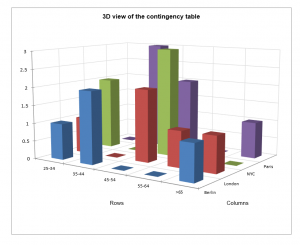Create contingency tables

Contingency tables are a way to visualize the relationship between two qualitative variables. Create them in Excel using the XLSTAT add-on software.What is a contingency table

A contingency table, also called cross-tab, is an efficient way to summarize the relation (or correspondence) between two categorical variables V1 and V2. It has the following structure:

 V1 V2 Category 1 Category j … Category m2 Category 1 n(1,1) n(1,j) … n(1,m2) … … … … … Category i n(i,1) n(i,j) … n(i,m2) … … … … … Category m1 n(m1,1) n(m1,j) … n(m1,m2)

where n(i,j) is the frequency of observations that show both characteristic i for variable V1, and characteristic j for variable V2.

To create a contingency table from two qualitative variables V1 and V2 , the first transformation consists of recoding the two qualitative variables V1 and V2 as two disjunctive tables Z1 and Z2 or indicator (or dummy) variables. For each category of a variable there is a column in the respective disjunctive table. Each time the category c of variable V1 occurs for an observation i , the value of Z1(i,c) is set to one (the same rule is applied to the V2 variable). The other values of Zand Z2 are zero. The contingency table of the two variables is the table Z1'Z2 (where ‘ indicates matrix transpose).

The Chi-square distance has been suggested to measure the distance between two categories. The Pearson chi-square statistic, which is the sum of the Chi-square distances, is used to test the independence between rows and columns. Is has asymptotically a Chi-square distribution with (m1-1)(m2-1) degrees of freedom.

Inertia is a measure inspired from physics that is often used in Correspondence Analysis, a method that is used to analyse in depth contingency tables. The inertia of a set of points is the weighted mean of the squared distances to the center of gravity.

XLSTAT tool for creating a contingency table

From two or more qualitative variables, XLSTAT enables you to create instantly contingency tables summarizing the structure of the dataset. The data should be selecting in the general tab of the tool using the below fields:

Row variable(s): Select the data that correspond to the variable(s) that will be used to construct the rows of the contingency table(s).

Column variable(s): Select the data that correspond to the variable(s) that will be used to construct the columns of the contingency table(s).

By group analysis: Activate this option and select the data that describe to which group each observation belongs. XLSTAT will then run the analysis for each group separately. Useful for creating three-way crosstabs.

Weights: Activate this option if the observations are weighted. If you do not activate this option, the weights will be considered as 1. Weights must be greater than or equal to 0. If a column header has been selected, check that the "Variable labels" option is activated.

XLSTAT tests on contingency tables

XLSTAT provides you with two criteria to characterize the relationship between the two variables:

• The Chi-square distance has been suggested to measure the distance between two categories. The Pearson chi-square statistic, which is the sum of the Chi-square distances, is used to test the independence between rows and columns.

The Chi-square by cell option available in the XLSTAT dialog box allows you to display the contribution to the chi-square of each cell of the contingency table.

• Inertia is a measure inspired from physics that is often used in Correspondence Analysis, a method that is used to analyse in depth contingency tables. The inertia of a set of points is the weighted mean of the squared distances to the center of gravity.

The Inertia by cell option available in the XLSTAT dialog box allows you to display the inertia for each cell of the contingency table.

You may also use a more complete feature dedicated to tests on contingency tables available in the Correlations/Association tests menu.

XLSTAT charts for contingency tables

3D view of the contingency table: Activate this option to display the 3D bar chart corresponding to the contingency table.

2D Bar Charts options

Chart type

• Grouped: Choose this option to display the graphs as bars grouped by modality.
• Stacked bars: Choose this option to display the chart as stacked bars. These charts are used to compare the frequencies of sub-samples to those of a full sample.

Bar charts

• Frequencies: Choose this option to display the frequencies corresponding to each bar.
• Percentages: Choose this option to display the % of population corresponding to each bar.

Among the many advantages of using the XLSTAT contingency table feature compared to Excel pivot tables:

• XLSTAT is able to automatically output test results on the contingency tables.
• You can enter as many qualitative variables as you want in both the row and column variable fields in XLSTAT. XLSTAT will produce one result for each possible pair of row/column variables.

Tutorial on how to create and interpret a contingency table

A tutorial which explains how to configure the XLSTAT dialog box in order to generate a cross-tab based on two qualitative variables is available.analice sus datos con xlstat

prueba gratuita de 14 días

Incluido en